## RS Aggarwal Class 8 Solutions Chapter 3 Squares and Square Roots Ex 3C

These Solutions are part of RS Aggarwal Solutions Class 8. Here we have given RS Aggarwal Solutions Class 8 Chapter 3 Squares and Square Roots Ex 3C.

Other Exercises

Question 1.
Solution:
(23)² = 529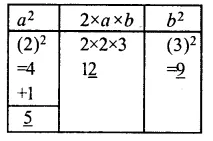Question 2.
Solution:
(35)² = 1225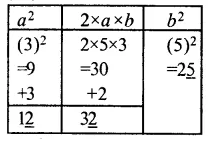Question 3.
Solution:
(52)² = 2704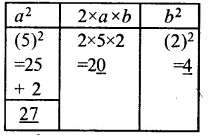Question 4.
Solution:
(96)² = 9216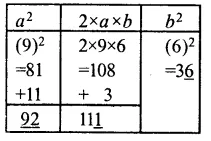Find the value of each of the following using the diagonal method :

Question 5.
Solution:
(67)² = 4489 Ans.Question 6.
Solution:
(86)² = 7396 AnsQuestion 7.
Solution:
(137)² = 18769 Ans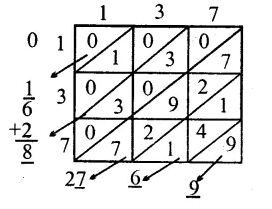Question 8.
Solution:
(256)² = 65536 Ans.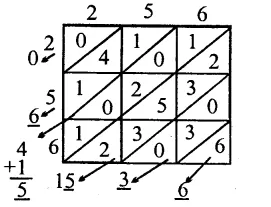Hope given RS Aggarwal Solutions Class 8 Chapter 3 Squares and Square Roots Ex 3C are helpful to complete your math homework.

If you have any doubts, please comment below. Learn Insta try to provide online math tutoring for you.